# Rectangular Prisms and Cubes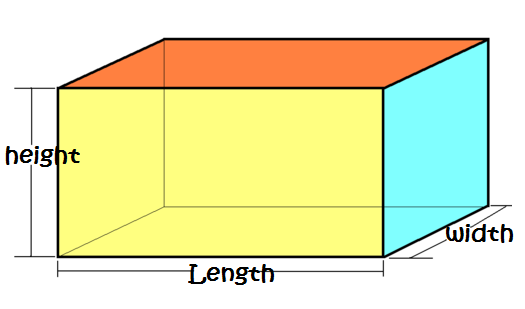A rectangular prism is a solid (3-dimensional) object that is shaped like a box.

It has 6 (flat) faces shaped like rectangles, 12 edges and 8 vertices (corners).

Every cross-section of a rectangular prism parallel to one of its sides is shaped like a rectangle.

Another name for a rectangular prism is a cuboid.

## Examples of Rectangular Prisms

Rectangular prisms are probably one of the most commonly found solid shapes in the world. We see them every morning when we eat our breakfast, we are very likely to sleep inside a rectangular prism, and we often store our belongings in rectangular prisms. Here are a few examples of some commonly seen rectangular prisms.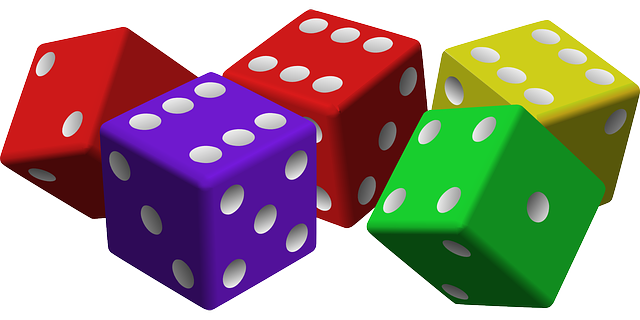We often play games with dice: rectangular prisms in which every face is a square.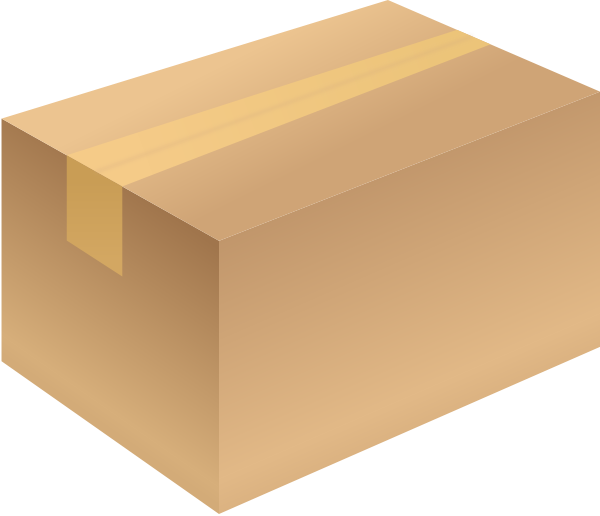Cardboard boxes are usually shaped like rectangular prisms.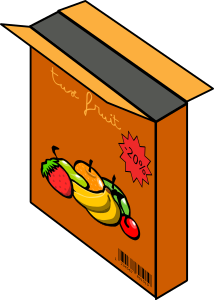Breakfast cereals come in boxes shaped like rectangular prisms.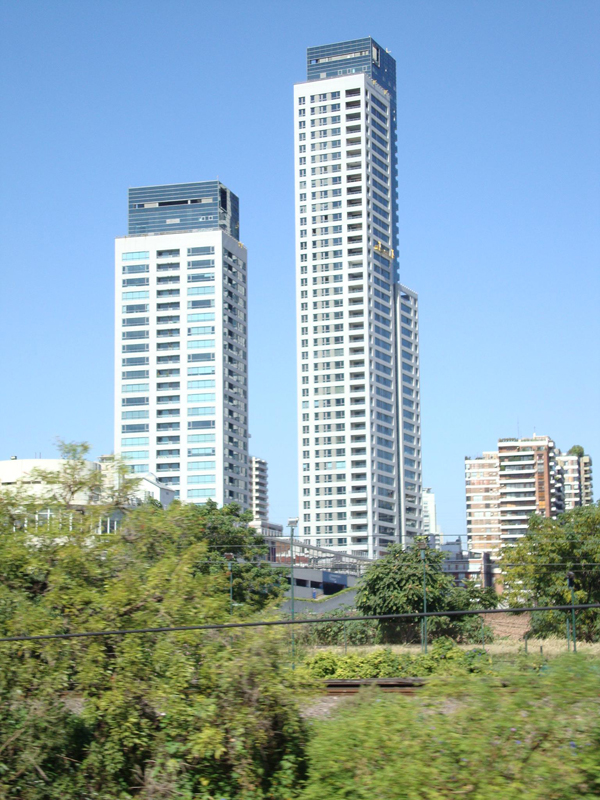Tall buildings are often shaped like rectangular prisms.

## Square Prisms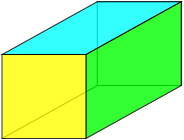A square prism is a rectangular prism in which two of the faces are squares (equal side-lengths).

## Cubes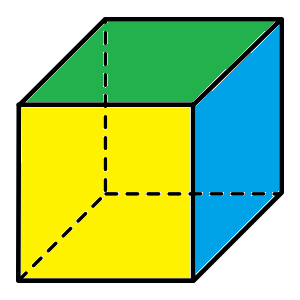Cubes are rectangular prisms in which every face is a square.

Another name for a cube is a hexahedron, and the cube is one of the Platonic solids.

Some people call rectangular prisms cuboids because they are shaped like cubes, except that all their side lengths are not equal.

## Surface Area of a Rectangular Prism

To find the surface area of a rectangular prism, we just add up the areas of all six of its faces.

The faces of a rectangular prism form three congruent pairs of rectangles, so we really only need to find the areas of three rectangles, and multiply themn by 2 as shown below.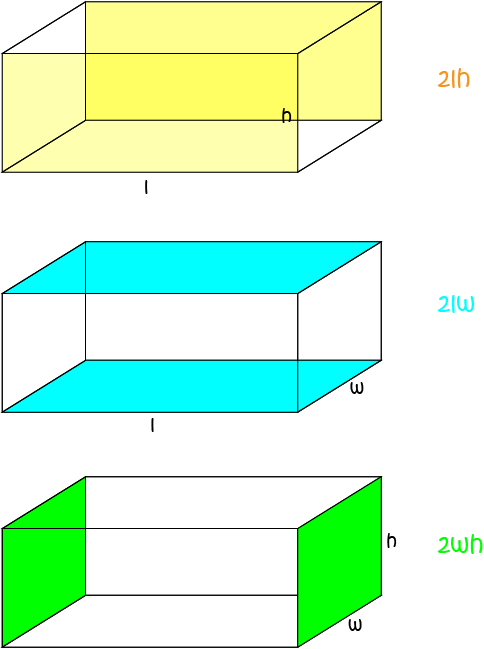The front and back faces are congruent rectangles of length $l$ and height $h$, so their total area is $2hl$.

The top and bottom faces are congruent rectangles of dimensions $w \times l$, so their total area is $2wl$.

The two end faces are congruent rectangles of dimensions $w \times h$, so their total area is $2wh$.

The surface area of the rectangular prism is the sum of the areas of these faces, or:

$A = 2 lh + 2 wl + 2 wh$

### Example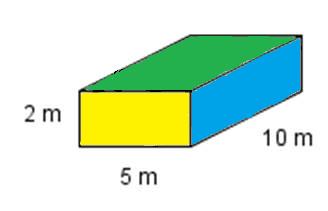Find the surface area of this rectangular prism.

Solution:

\begin{align*} A &= 2 lh + 2 wl + 2 wh\\ &= 2 \times 10 \times 2 + 2 \times 5 \times 10 + 2 \times 2 \times 5\\ &= 40 + 100 + 20\\ &= 160 \text{ m}^2 \end{align*}

## Volume of a Rectangular Prism

The volume of a rectangular prism is given by the formula

$\text{Volume} = \text{length} \times \text{width} \times \text{height}$
or
$V = lwh$
for short.

### ExampleFind the volume of this rectangular prism.

Solution:

\begin{align*} V &= lwh\\ &= 10 \times 5 \times 2\\ &= 100 \text{ m}^3 \end{align*}

### Description

There are several lessons related to 3D geometry such as

1. Euler's formula
2. Vertices, Edges and Faces
3. Volumes of 3D shapes
4. etc

Even though we've titled this lesson series to be more inclined for Year 10 or higher students, however, these lessons can be read and utilized by lower grades students.

### Prerequisites

Understanding of 3D shapes

### Audience

Year 10 or higher, but suitable for Year 8+ students

### Learning Objectives

Get to know 3D Geometry

Author: Subject Coach
You must be logged in as Student to ask a Question.